# Coauthorships in Network Science Network

Weighted network of coauthorships between scientists working on network science

Weighted network of coauthorships between scientists working on network theory and experiment with edge weight indicating the strength of the collaboration between pairs of individuals.

## Examples

### Basic Examples

Retrieve the graph:

 In:=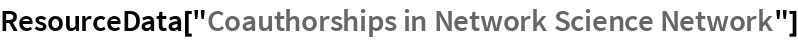Out=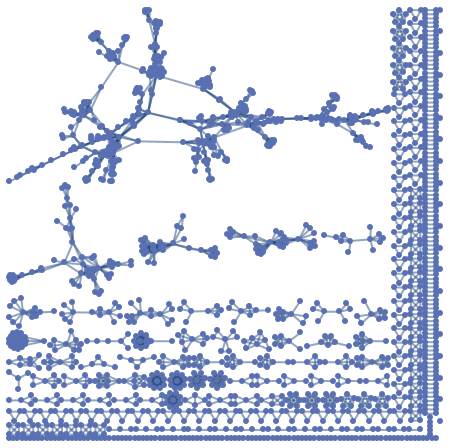Summary properties:

 In:=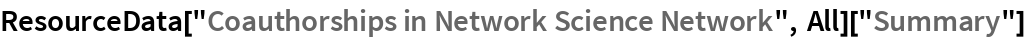Out=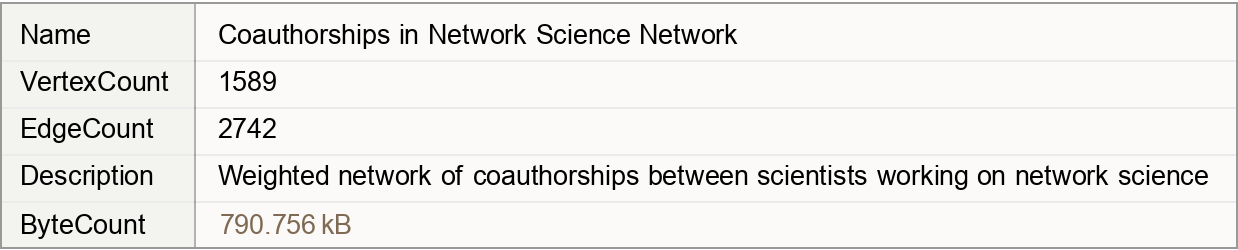### Basic Applications

Pick the author with the most collaborations:

 In:=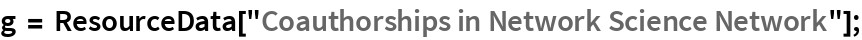In:=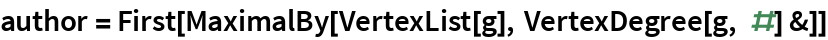Out=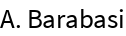Visualize the network around the author with the most collaborations:

 In:=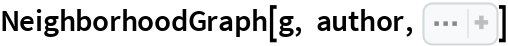Out=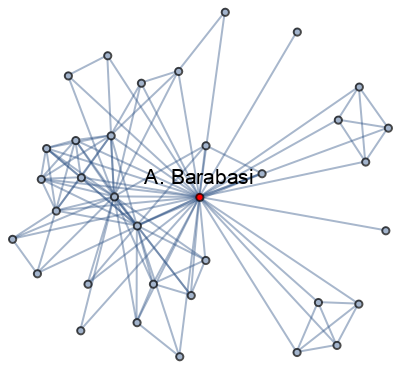Wolfram Research, "Coauthorships in Network Science Network" from the Wolfram Data Repository (2019)